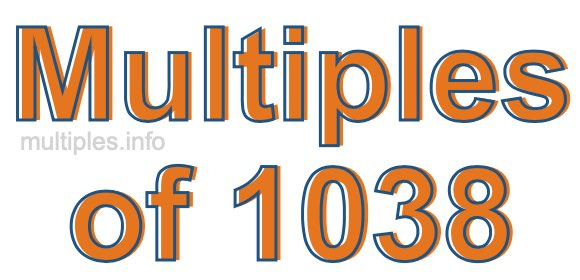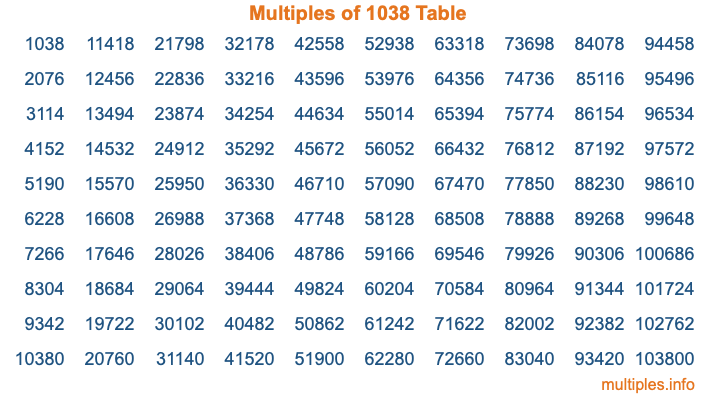Multiples of 1038Welcome to the Multiples of 1038 page. Here we will first teach you everything you will ever need to know about the multiples of 1038, and then give you a study guide summary of everything we taught you to make sure you remember it all. Use this page to look up facts and learn information about the multiples of 1038. This page will make you a multiples of one thousand thirty-eight expert!

Definition of Multiples of 1038
Multiples of 1038 are all the numbers that when divided by 1038 equal an integer. Each of the multiples of 1038 are called a multiple. A multiple of 1038 is created by multiplying 1038 by an integer.

Therefore, to create a list of multiples of 1038, you start with 1 multiplied by 1038, then 2 multiplied by 1038, then 3 multiplied by 1038, and so on for as long as you want. Thus, the list of the first five multiples of 1038 is 1038, 2076, 3114, 4152, and 5190. To see a larger list of multiples of 1038, see the printable image of Multiples of 1038 further down on this page. We also have a category where you can choose any nth multiple of 1038.

Multiples of 1038 Checker
The Multiples of 1038 Checker below checks to see if any number of your choice is a multiple of 1038. In other words, it checks to see if there is any number (integer) that when multiplied by 1038 will equal your number. To do that, we divide your number by 1038. If the the quotient is an integer, then your number is a multiple of 1038.

Is  a multiple of 1038?

Least Common Multiple of 1038 and ...
A Least Common Multiple (LCM) is the lowest multiple that two or more numbers have in common. This is also called the smallest common multiple or lowest common multiple and is useful to know when you are adding our subtracting fractions. Enter one or more numbers below (1038 is already entered) to find the LCM.

Check out our LCM Calculator if you need more details about the Least Common Multiple or if you need the LCM for different numbers for adding and subtraction fractions.

nth Multiple of 1038
As we stated above, 1038 is the first multiple of 1038, 2076 is the second multiple of 1038, 3114 is the third multiple of 1038, and so on. Enter a number below to find the nth multiple of 1038.

th multiple of 1038

Multiples of 1038 vs Factors of 1038
1038 is a multiple of 1038 and a factor of 1038, but that is where the similarities end. All postive multiples of 1038 are 1038 or greater than 1038. All positive factors of 1038 are 1038 or less than 1038.

Below is the beginning list of multiples of 1038 and the factors of 1038 so you can compare:

Multiples of 1038: 1038, 2076, 3114, 4152, 5190, etc.

Factors of 1038: 1, 2, 3, 6, 173, 346, 519, 1038

As you can see, the multiples of 1038 are all the numbers that you can divide by 1038 to get a whole number. The factors of 1038, on the other hand, are all the whole numbers that you can multiply by another whole number to get 1038.

It's also interesting to note that if a number (x) is a factor of 1038, then 1038 will also be a multiple of that number (x).

Multiples of 1038 vs Divisors of 1038
The divisors of 1038 are all the integers that 1038 can be divided by evenly. Below is a list of the divisors of 1038.

Divisors of 1038: 1, 2, 3, 6, 173, 346, 519, 1038

The interesting thing to note here is that if you take any multiple of 1038 and divide it by a divisor of 1038, you will see that the quotient is an integer.

Multiples of 1038 Table
Below is an image of the first 100 multiples of 1038 in a table. The table is in chronological order, column by column. The first column has the first ten multiples of 1038, the second column has the next ten multiples of 1038, and so on.The Multiples of 1038 Table is also referred to as the 1038 Times Table or Times Table of 1038. You are welcome to print out our table for your studies.

Negative Multiples of 1038
Although not often discussed or needed in math, it is worth mentioning that you can make a list of negative multiples of 1038 by multiplying 1038 by -1, then by -2, then by -3, and so on, to get the following list of negative multiples of 1038:

-1038, -2076, -3114, -4152, -5190, etc.

Multiples of 1038 Summary
Below is a summary of important Multiples of 1038 facts that we have discussed on this page. To retain the knowledge on this page, we recommend that you read through the summary and explain to yourself or a study partner why they hold true.

There are an infinite number of multiples of 1038.

A multiple of 1038 divided by 1038 will equal a whole number.

1038 divided by a factor of 1038 equals a divisor of 1038.

The nth multiple of 1038 is n times 1038.

The largest factor of 1038 is equal to the first positive multiple of 1038.

1038 is a multiple of every factor of 1038.

1038 is a multiple of 1038.

A multiple of 1038 divided by a divisor of 1038 equals an integer.

1038 divided by a divisor of 1038 equals a factor of 1038.

Any integer times 1038 will equal a multiple of 1038.

Multiples of a Number
Here you can get the multiples of another number, all with the same attention to detail as we did for multiples of 1038 on this page.

Multiples of
Multiples of 1039
Did you find our page about multiples of one thousand thirty-eight educational? Do you want more knowledge? Check out the multiples of the next number on our list!